# Basic Arithmetic : Changing a Fraction to Percent

## Example Questions

### Example Question #33 : Percents And Decimals

Ifstudents out of a class ofare wearing blue, what percentage of the class is NOT wearing blue?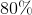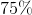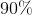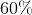Explanation:

Ifof the students are wearing blue then that meansof them are not wearing blue. Because,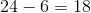Now to find the percentage we divide the number of students not wearing blue, by the total number of students in the class and then multiply by.

Thus,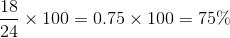### Example Question #1 : Changing A Fraction To Percent

Convert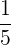to a percentage.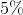Explanation:

The correct answer is 20%. This can be obtained by first converting the fractionto a decimal and then multiplying by 100 to get a percentage.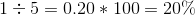### Example Question #11 : Changing View

Change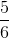to a percent.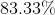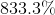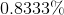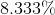Explanation:

To convert a fraction into a percent, we need to first change the fraction into a decimal.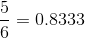Now, change the decimal into a percent by multiplying by 100 and adding a percent sign.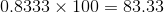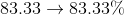### Example Question #12 : Changing View

What is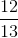as a percent?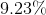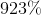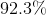Explanation:

To change a fraction into a percent, you need to first change the fraction into a decimal.

Dividing 12 by 13 gives the following: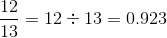Now, to change the decimal to a percent, start by multiplying the decimal by 100.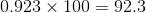Then, just add a percent sign.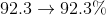### Example Question #13 : Changing View

What is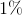of?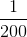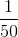Explanation:

Convert 1% to a fraction by dividing 100.  One percent is equivalent to: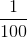Multiply this with two, and the answer is: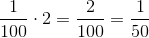### Example Question #14 : Changing View

Convert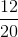to a percent.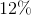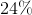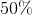Explanation:

A percentage can be written as a fraction with any positive whole number in the numerator and 100 in the denominator. To write the fraction as a percent, begin by writing both as fractions: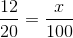Cross-multiply to find the missing whole number.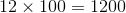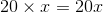Divide the known value by the unknown to solve for x.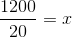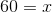ispercent of, so the answer is.

### All Basic Arithmetic Resources